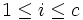# Supersolvable implies nilpotent derived subgroup

This article gives the statement and possibly, proof, of an implication relation between two group properties. That is, it states that every group satisfying the first group property (i.e., supersolvable group) must also satisfy the second group property (i.e., group with nilpotent derived subgroup)
View all group property implications | View all group property non-implications
Get more facts about supersolvable group|Get more facts about group with nilpotent derived subgroup

## Statement

The derived subgroup of a supersolvable group is a nilpotent group. Moreover, the nilpotency class of the derived subgroup is bounded from above by the length of any normal series for the whole group where each of the quotient groups between successive members is a cyclic group.

## Facts used

1. Derived subgroup centralizes cyclic normal subgroup
2. Derived subgroup is normal
3. Normality is strongly intersection-closed
4. Normality satisfies image condition
5. Second isomorphism theorem
6. Cyclicity is subgroup-closed
7. Derived subgroup satisfies image condition: Under a surjective homomorphism, the image of the derived subgroup equals the derived subgroup of the image.

## Proof

This proof uses a tabular format for presentation. Provide feedback on tabular proof formats in a survey (opens in new window/tab) | Learn more about tabular proof formats|View all pages on facts with proofs in tabular format

Given: A supersolvable group$G$ with a normal series$1 = N_0 \le N_1 \le \dots N_c = G$ with$N_i/N_{i-1}$ cyclic.

To prove:$[G,G]$ is nilpotent of class at most$c$.

Proof: We will prove that the series:$1 = N_0 \cap [G,G] \le N_1 \cap [G,G] \le \dots \le N_c \cap [G,G] = [G,G]$

is a central series for$[G,G]$.

Step no. Assertion/construction Facts used Given data used Previous steps used Explanation
1$[G,G]$ is normal in$G$ Fact (2)
2$N_i \cap [G,G]$ is normal in$G$ for all$i$ Fact (3)$N_i$ are all normal in$G$ Step (1)
3$(N_i \cap [G,G])/(N_{i-1} \cap [G,G])$ is normal in$G/(N_{i-1} \cap [G,G])$ for all$1 \le i \le c$ Fact (4)$N_i$ are all normal in$G$ Step (2) (applied to both$i$ and$i - 1$)
4$(N_i \cap [G,G])/(N_{i-1} \cap [G,G])$ is cyclic Fact (6)$N_i/N_{i-1}$ is cyclic Step (2) (applied to$i-1$) By the second isomorphism theorem (fact (5)), this quotient is isomorphic to$N_{i-1}(N_i \cap [G,G])/N_{i-1}$, which in turn is a subgroup of$N_i/N_{i-1}$. Fact (6) therefore yields that it is cyclic.
5$[G,G]/(N_{i-1} \cap [G,G])$ is the derived subgroup of$G/(N_{i-1} \cap [G,G])$ Fact (7)
6$(N_i \cap [G,G])/(N_{i-1} \cap [G,G])$ is in the center of$[G,G]/(N_{i-1} \cap [G,G])$ Fact (1) Steps (3), (4), (5)$(N_i \cap [G,G])/(N_{i-1} \cap [G,G])$ is cyclic normal in$G/(N_{i-1} \cap [G,G])$, and$[G,G]/(N_{i-1} \cap [G,G])$ is the derived subgroup. So fact (1) yields that$(N_i \cap [G,G])/(N_{i-1} \cap [G,G])$ is in the center of$[G,G]/(N_{i-1} \cap [G,G])$.
7 The series is indeed a central series. Step (6) (in light of step (2))